Measuring Length

Measuring Length

In this mini-lesson, we will learn about measuring length by understanding different units to measure length, how to compare length, and how to apply them while solving problems. We will also discover interesting facts around them.

Have you ever measured the length of your study table with your hand?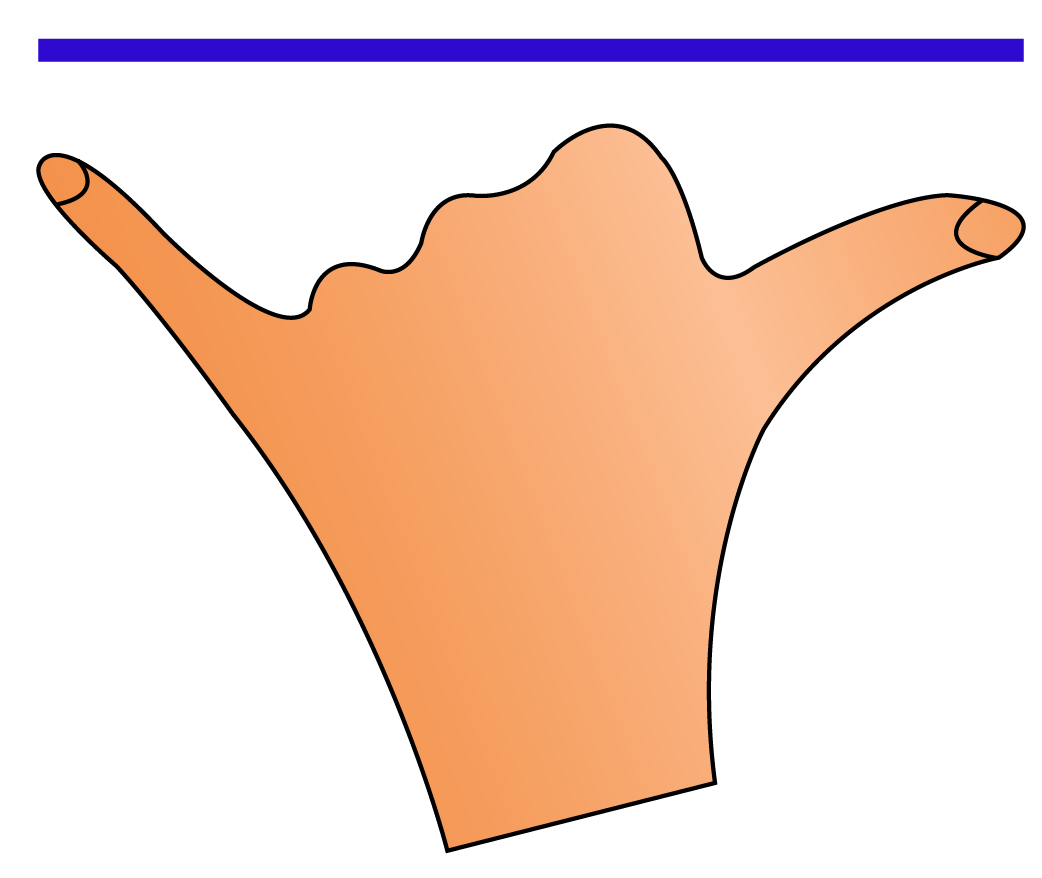Or have you compared the heights of you and your friend with the help of a rope piece?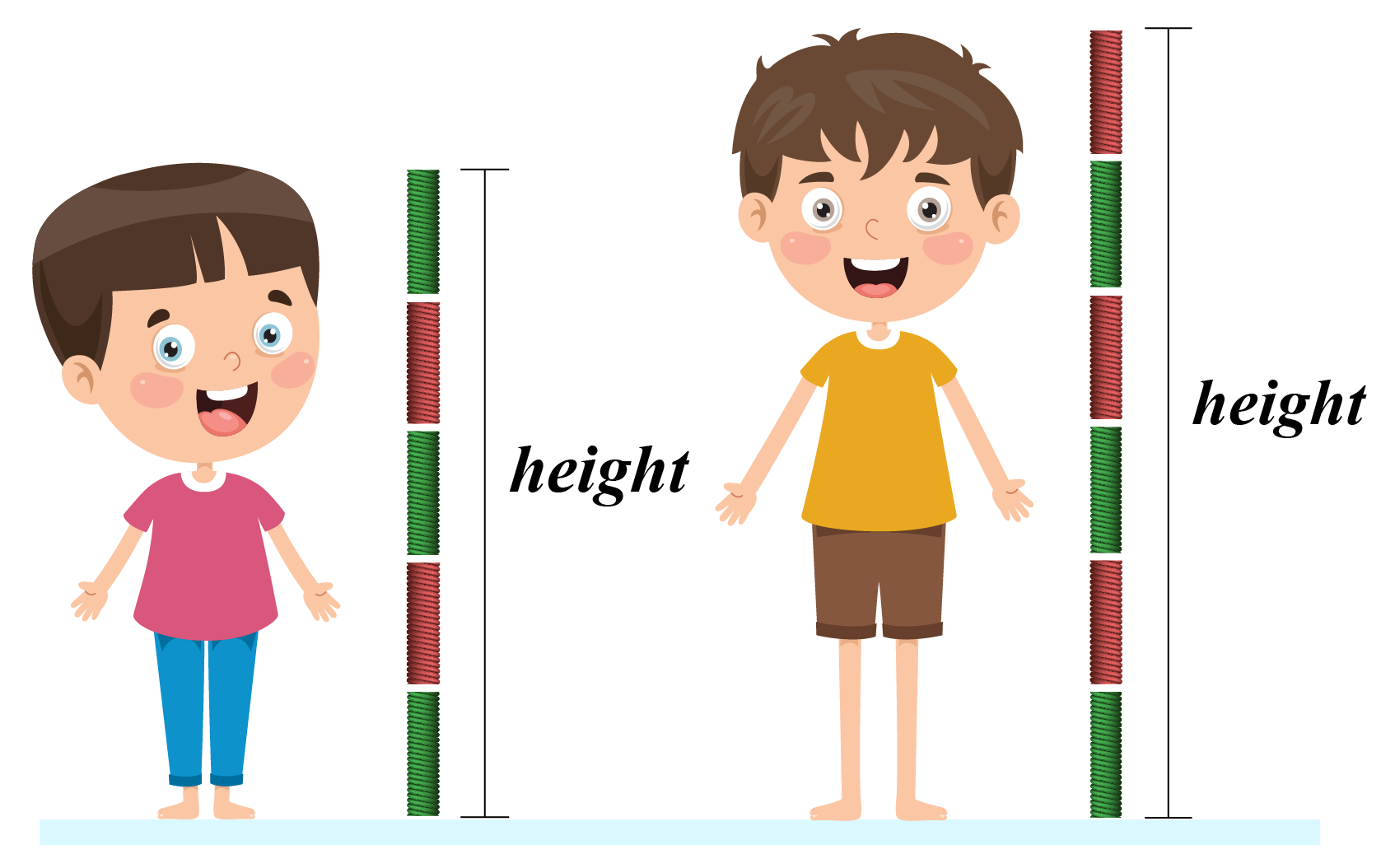We measure the length of various objects around us with the help of tools like scale, hand span, ropes, and many more.

let's explore the unit system for measuring lengths and how can we compare the lengths of two objects in this session.

Lesson Plan

 1 What Is Measuring Length? 2 Thinking Out of the Box! 3 Important Notes on Measuring Length 4 Solved Examples on Measuring Length 5 Interactive Questions on Measuring length

What Is Measuring Length?

Measuring the lengths of objects is a part of our day to day life, we always performed these type of tasks in which we measure the length.

Measuring length means to measure the length of any object with the help of measuring tools like scale.

For example, the length of a pencil can be measured in inches using a ruler.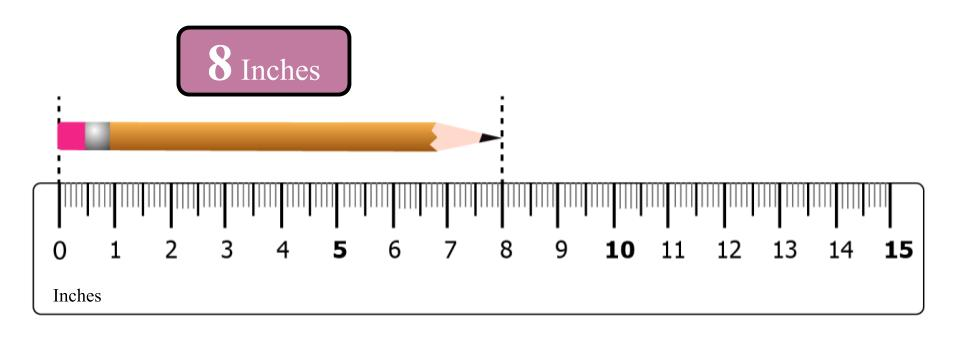The height of students in a class can be measured using a feet scale.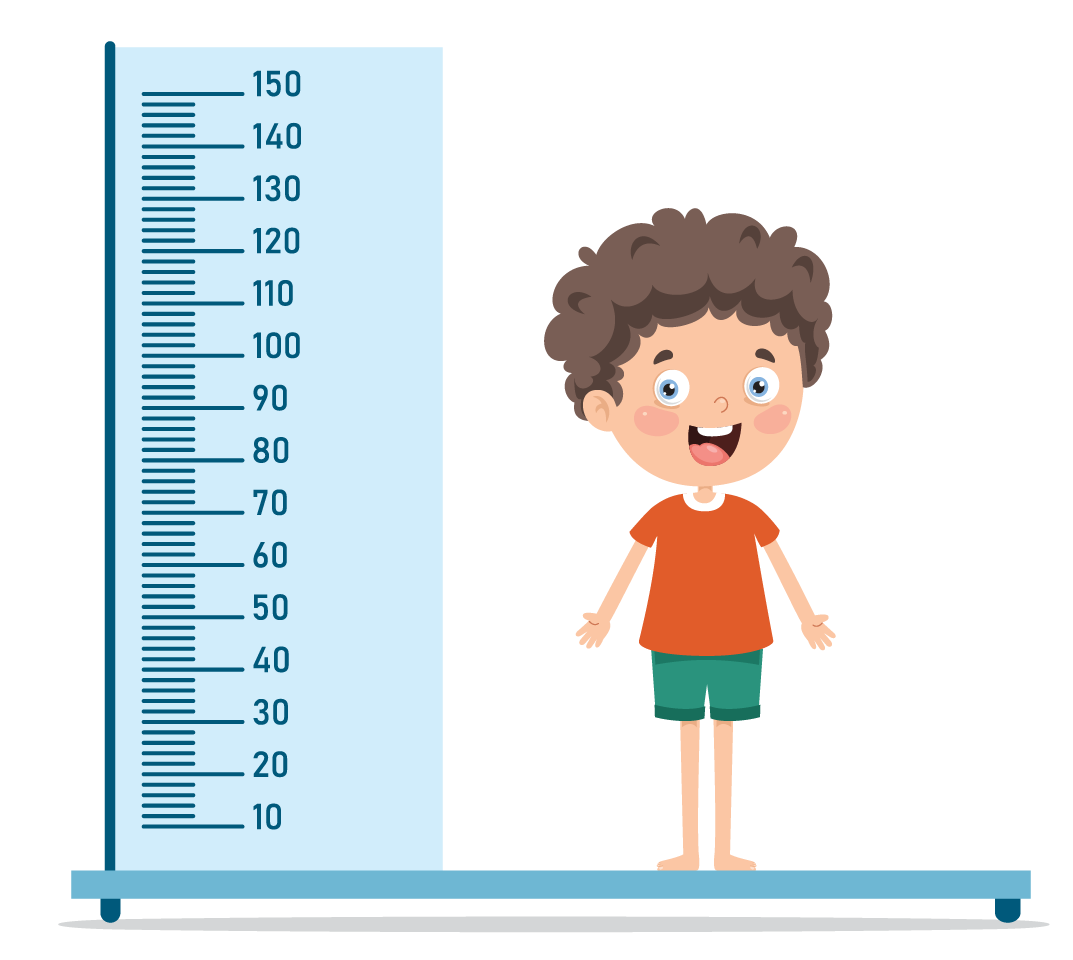Think Tank
• If the heights of all of your friends are arranged in ascending order then find out who is the tallest and who is the smallest.
• If the radius of an electron is $$10^{-18}$$ m, how many electrons will be grouped together to form a vertical line equal to your height?

What Units Do We Use to Measure Length?

We can use different units to measure the lengths of any object, these units are called imperial measurement units.

Imperial Measurement Units

The imperial system of units is the system of units first defined by the British, to measure different quantities.

The Weights and Measures Act of 1824 and the Act of 1878 established the British Imperial System on the basis of precise definitions of selected existing units.

Some commonly used units in the imperial measurement systems are.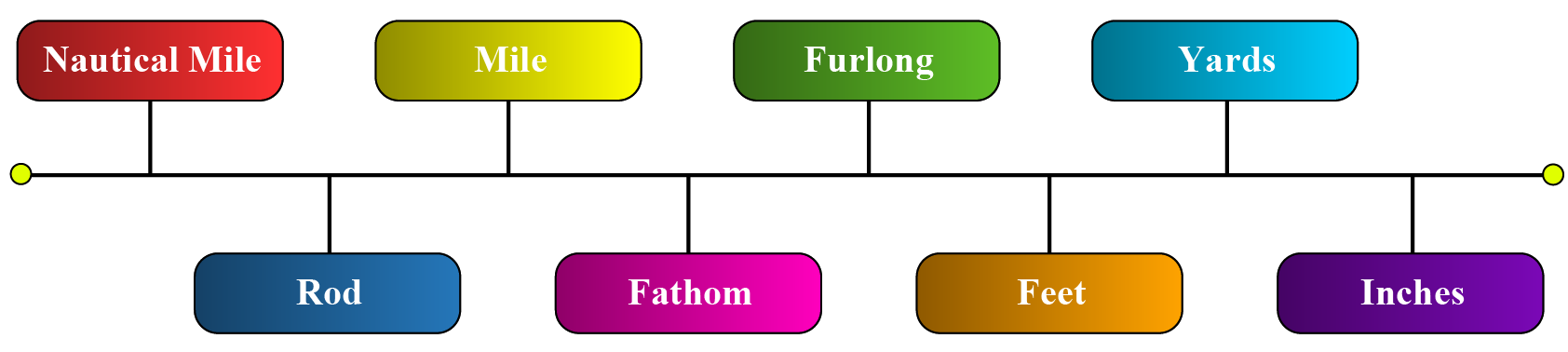Let us see the unit conversion table for the imperial measurement system of length.

 1 nautical mile 1.151 miles 1852 meters 1 mile 1760 yards 1609 meters 1 furlong 220 yards 201 meters 1 rod 5.50 yards 5.029 meters 1 fathom 6 feets 1.829 meters 1 yard 3 feets 0.9144 meters 1 foot 12 inches 0.3048 meters 1 inch 0.083 foot 0.0254 meters

How Do We Compare Lengths?

We can easily compare the lengths of two or more objects by simply looking at them, let's see a few examples.

We can tell by looking at the pictures of two ropes, which one is large and which is small.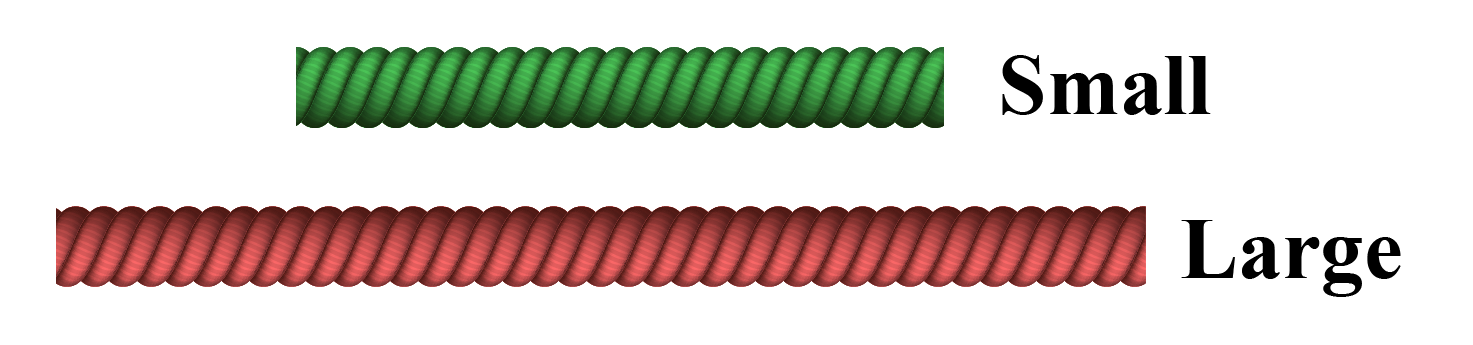We can clearly say that the red rope is larger than the green rope.

Now let's take two buildings and see which one is taller.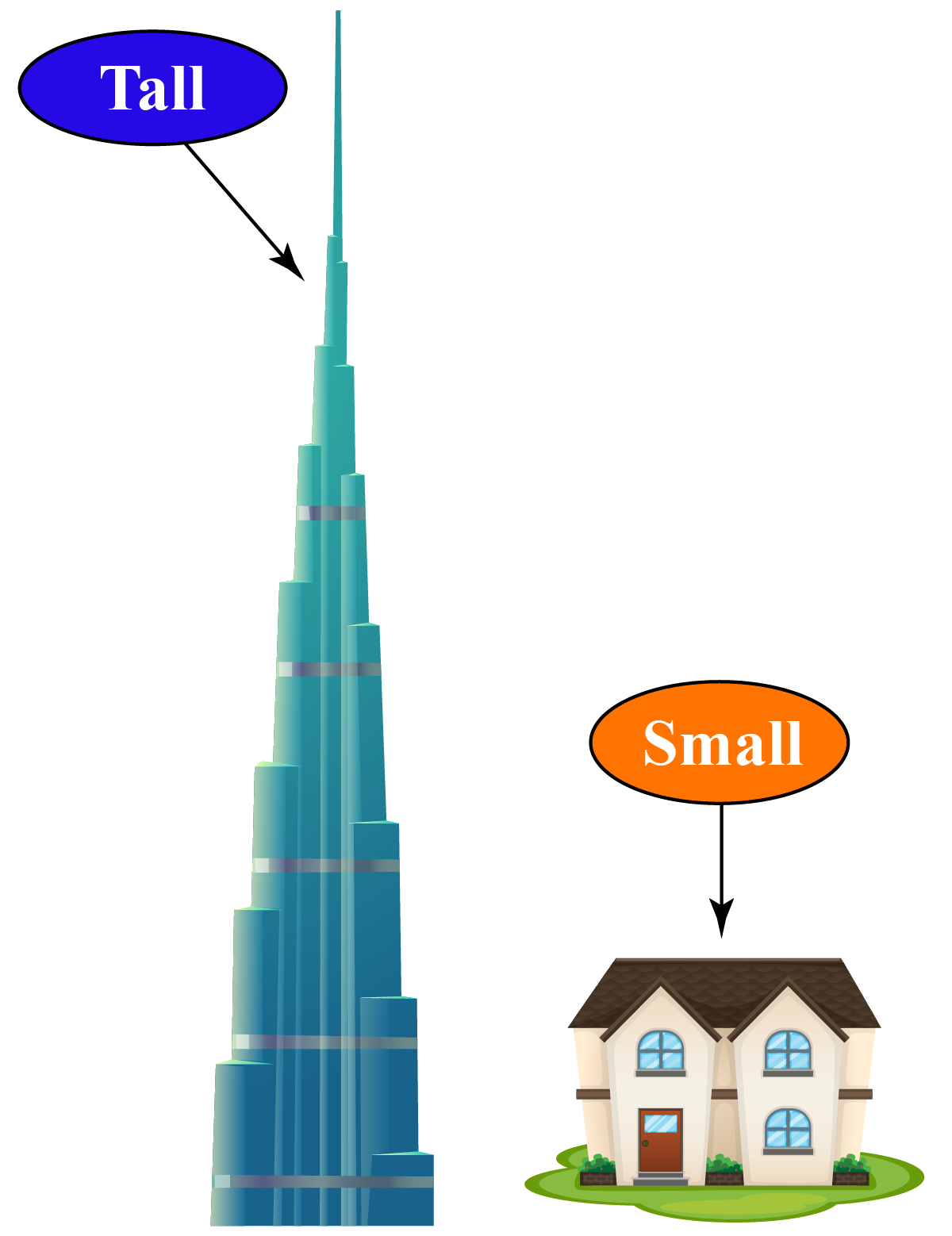Burj Khalifa is much taller than the house.

Let's consider Sam and his father, can we tell who is taller?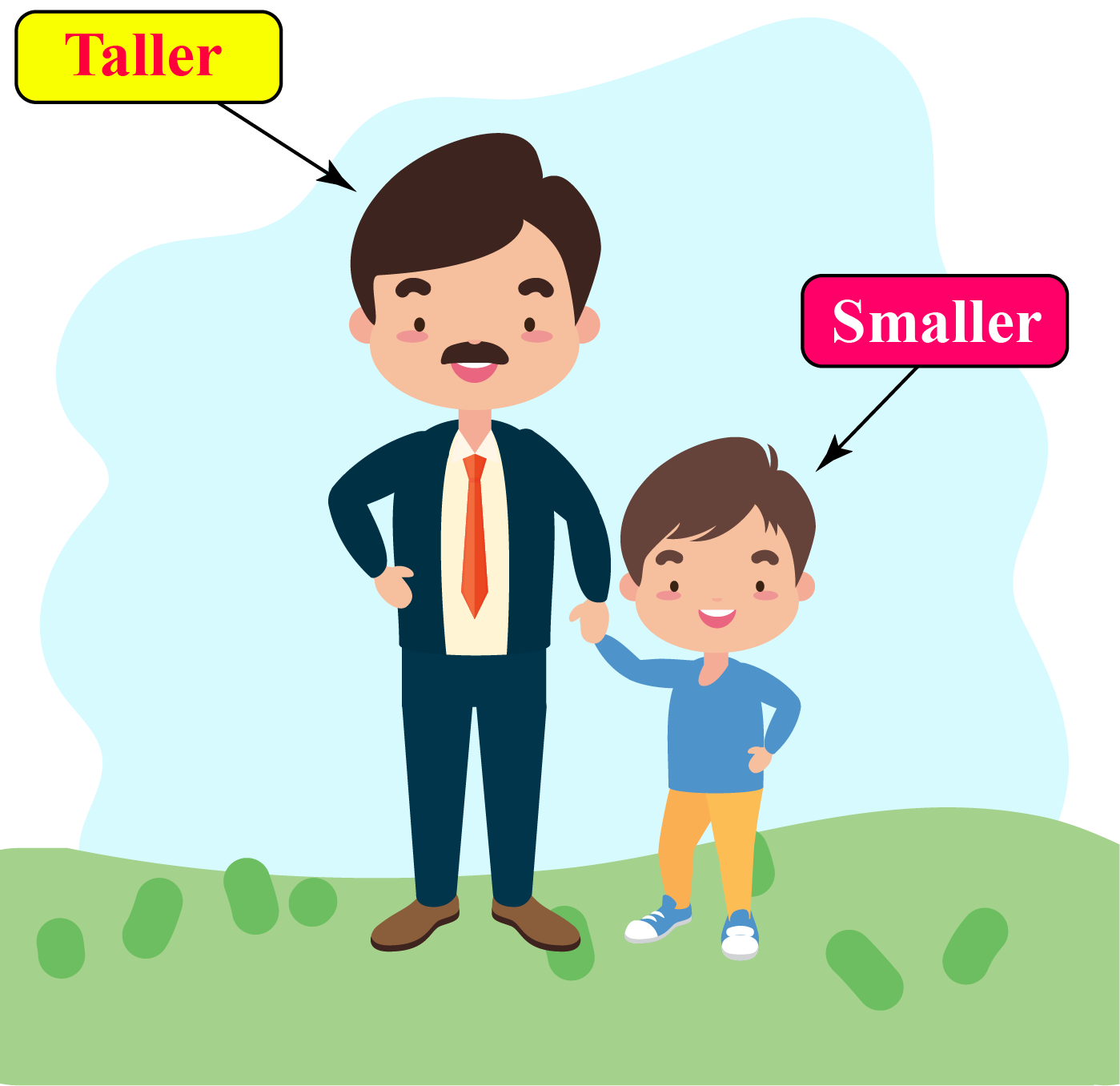We can clearly see that the father is taller than Sam.

What about an elephant and a lion?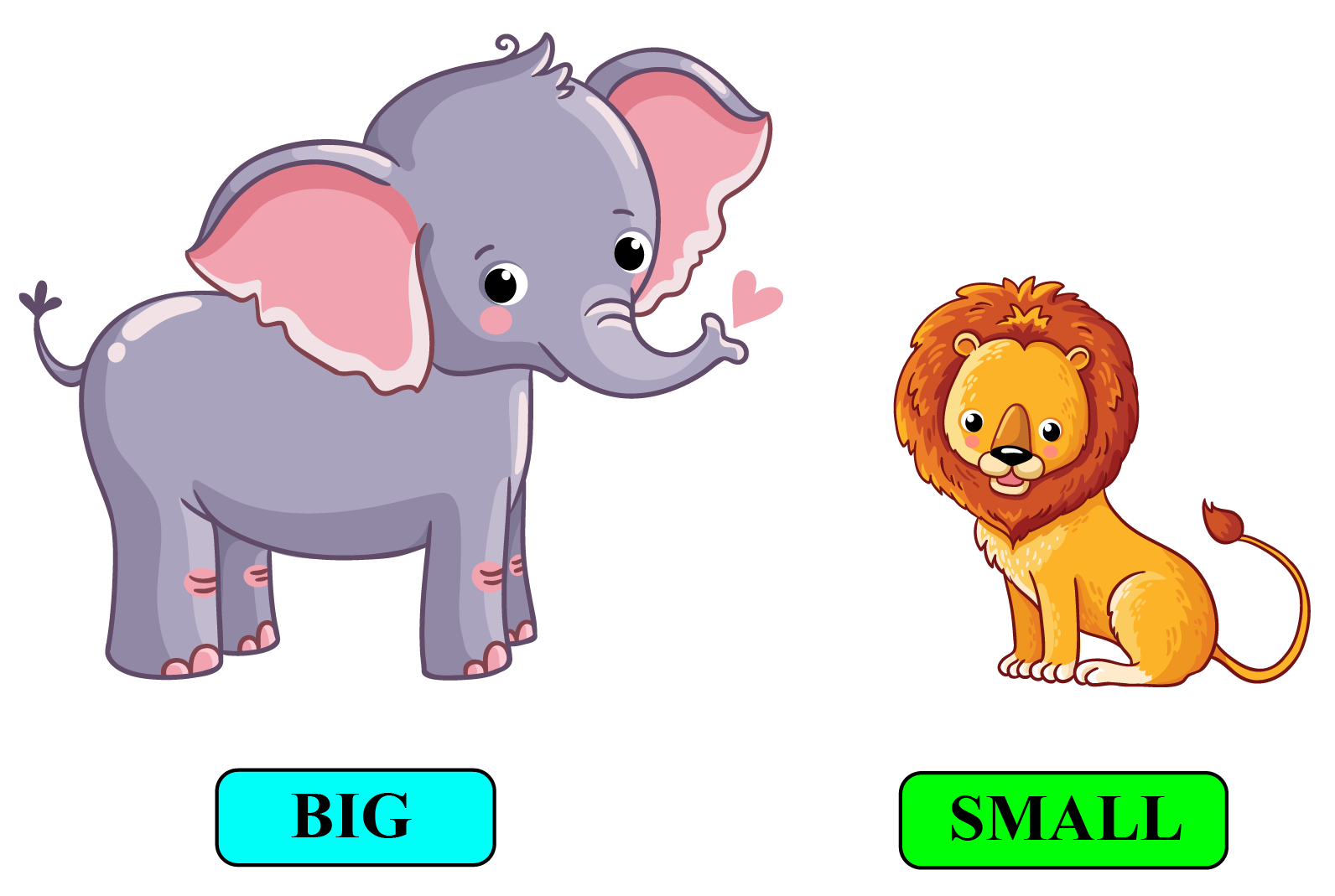We can clearly see that the elephant is bigger than the lion.

 Example 1

If the height of Peter's father is 50% more than the height of Peter. If Peter is 52 inches then the height of the father is?

Solution

Given the height of Peter = 52 inches

Height of father is 50% more

\begin{align} \text{Height of father} &= \text{52 + 50% of 52} \\[0.2cm] \text{Height of father} &= \text{52} + \dfrac{1}{2} \times 52 \\[0.2cm] \text{Height of father} &= \text{52 + 26} \\[0.2cm] \text{Height of father} &= \text{78 inches} \\[0.2cm] \end{align}

 $$\therefore$$ Height of father is 78 inches.
 Example 2

If James covers 0.5 yards in one step then how much distance will he cover in 50 steps?

Solution

Distance covered in one step = 0.5 yards

Total steps taken by James = 50

Total distance covered by James = $$0.5 \times 50 = 25 \text{ yards}$$

 $$\therefore$$ James covered 25 yards.
 Example 3

A rectangular field has to be fenced by single iron rope, if the measurement of the length of the field is 100 yards and the breadth is 80 yards, how much fencing rope will be required?

Solution

Dimensions of the rectangular field are given as:

Length = 100 yards

Width = 80 yards

Perimeter of the field = $$2 (100 + 80)$$

Perimeter of the field = $$2 \times 180 = 360 \text{ yards}$$

 $$\therefore$$ Length of the rope required is 360 yards

Interactive Questions on Measuring Length

Here are a few activities for you to practice. Select/Type your answer and click the "Check Answer" button to see the result.

Let's Summarize

This mini-lesson introduced you to the fascinating concept of measuring length. The math journey around measuring length starts with what a student already knows, and goes on to creatively crafting a fresh concept in the young minds. Done in a way that is not only relatable and easy to grasp, but also will stay with them forever. You have learned about measuring length, units to measure length, and comparison of length in this lesson. Here lies the magic with Cuemath.

At Cuemath, our team of math experts is dedicated to making learning fun for our favorite readers, the students! Through an interactive and engaging learning-teaching-learning approach, the teachers explore all angles of a topic. Be it simulations, online classes, doubt sessions, or any other form of relation, it’s the logical thinking and smart learning approach that we at Cuemath believe in.

1. What is the standard unit of measuring length?

The standard unit of measuring length is a meter.

2. How to measure the length with a ruler?

The length of any object can be measured with help of a ruler easily, just place the ruler beside the object and measure the readings from the beginning of the object to the end of the object on the scale.

A Visual Introduction to Measurement
Kg | Worksheet 1
A Visual Introduction to Measurement
Kg | Worksheet 2
More Important Topics
Numbers
Algebra
Geometry
Measurement
Money
Data
Trigonometry
Calculus
More Important Topics
Numbers
Algebra
Geometry
Measurement
Money
Data
Trigonometry
Calculus
Learn from the best math teachers and top your exams

• Live one on one classroom and doubt clearing
• Practice worksheets in and after class for conceptual clarity
• Personalized curriculum to keep up with school